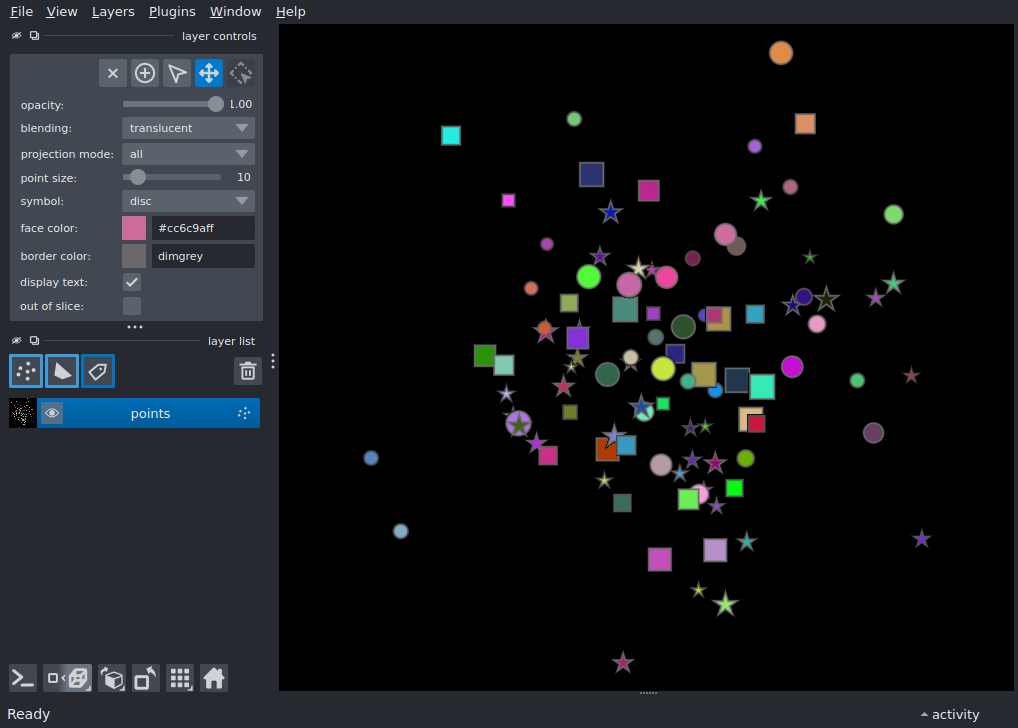# Point cloud¶

Display 3D points with combinations of different renderings.

Tags: visualization-basic```import numpy as np

import napari

n_points = 100

points = np.random.normal(10, 100, (n_points, 3))
symbols = np.random.choice(['o', 's', '*'], n_points)
sizes = np.random.rand(n_points) * 10 + 10
colors = np.random.rand(n_points, 3)

viewer = napari.Viewer(ndisplay=3)